Vibrations

# Vibrations - Mechanical Engineering SSC JE (Technical)

VIBRATIONS

Any motion that exactly repeats itself after a certain interval of time is a periodic motion . A periodic motion which is having to and fro motion is called vibration.

TYPE OF VIBRATION

•  Free Vibrations : Vibrations in which there are no friction and external force after the initial release of the body, are known as free or natural vibrations.
•  Forced Vibrations : When a repeated force continuously acts on a system, the vibrations are said to be forced vibration. The frequency of the vibrations depends upon the applied force and is independent of there own natural frequency of vibrators.
•  Damped Vibrations : When the energy of a vibrating system is gradually dissipated by friction and other resistances, the vibrations are said to be damped vibration.
•  Undamped Vibrations (Hypothetical) :When there is no friction or resistance present in system to counter act vibration then body execute undamped vibration.
•  Longitudinal Vibrations : If the shaft is elongated and shortened so that the same moves up and down resulting in tensile and compressive stresses in the shaft, the vibrations are said to be longitudinal.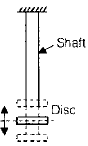•  Transverse Vibrations : When the shaft is bent alternately and tensile and compressive stresses due to bending result, the vibrations are said to be transverse vibration.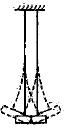•  Torsional Vibrations :When the shaft is twisted and untwisted alternately and torsional shear stresses are induced the vibrations are known as torsional vibrations.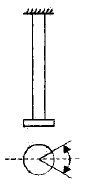BASIC ELEMENT OF VIBRATING SYSTEM

•   Inertial element : These are represented by lumped masses for rectilinear motion and by lumped moment of inertia for angular motion.
•   Restoring Elements : Massless linear or torsional springs represent the restoring elements for rectilinear and torsional motions respectively.
•  Damping Elements : Massless dampers of rigid elements may be considered for energy dissipation in a system.

FREE LONGITUDINAL VIBRATION
Different methods for finding natural frequency of a vibrating system
(i) Equilibrium method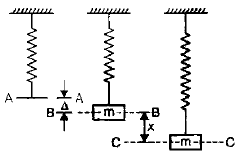•  It is based on the principle that whenever a vibratory system is in equilibrium the algebraic sum of forces and moments acting on it is zero.
•  This is in accordance with D' Alembert's principle that the sum of the inertial forces and the external forces on a body in equilibrium must be zero.

Let, D = static deflection
S = stiffness of the spring
Inertia force = m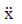(upwards) = ma, where a =Spring force = sx (upwards)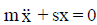This is the equation of a simple harmonic motion and is analogous to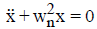•  The solution of which is given by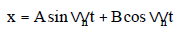where A and B are the constants of integration and their values depend upon the manner in which the vibration starts.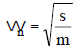•  Linear frequency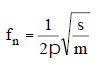Time period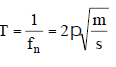(ii) Energy Method
• In a conservative system (system with no damping) the total mechanical energy i.e. the sum of the kinetic and the potential energies remains constant.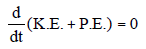We know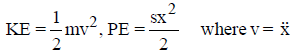where v =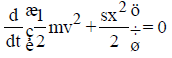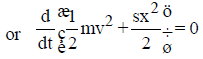or ma + sx = 0(iii) Rayleigh's Method

•  In this method, the maximum kinetic energy at the mean position is made equal to the maximum potential energy (or strain energy) of the extreme position.

Let the motion be simple harmonic
therefore, x = Xsinwnt
where X = maximum displacement from the mean position of the extreme position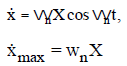or KE at mean position = PE at extreme position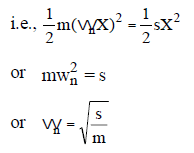•  Inertial effect of mass of spring

If we consider mass of spring is 'm1' then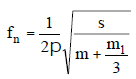DAMPED LONGITUDINAL VIBRATION

•  When an elastic body is set in vibratory motion, the vibrations die out after some  time due to the internal molecular friction of the mass of the body and the friction of  the medium in which it vibrates. The diminishing of vibrations with time is called  damping.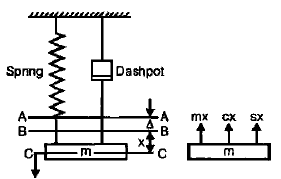Assumption
• The damping force is proportional to the velocity of vibration at lower values of speed and proportional to the square of velocity at higher speeds.
Let, s = stiffness of the spring
c = damping coefficient (damping force per unit velocity)
wn = frequency of natural undamped vibrations
x = displacement of mass from mean position at time 't'
v =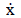= velocity of the mass at time 't'
f == acceleration of the mass at time 't'.
As the sum of the inertial force and the external forces on a body in any direction is
to be zero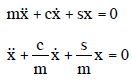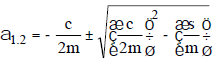Damping factor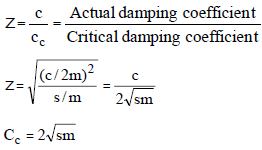Points to Rememberz = 1, the damping is criticalz > 1, the system is over-dampedz < 1, the system is under-damped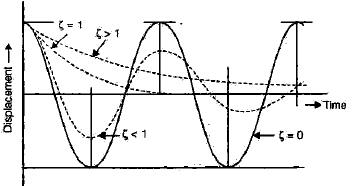Logarithmic Decrement (d) : The ratio of two successive oscillations is constant in an underdamped system. Natural logarithm of this ratio is called logarithmic decrement.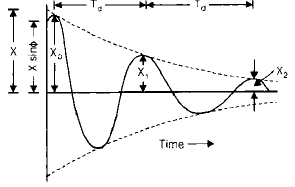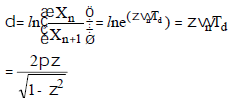FORCED VIBRATION

•  Step-input force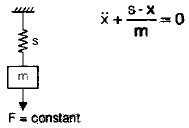•  Harmonic force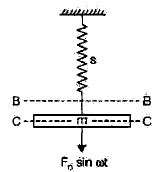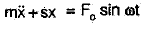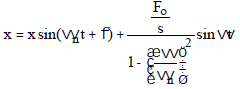FORCED DAMPED VIBRATIONS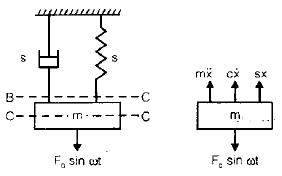If the mass is subjected to an oscillating force F = F0 sinwt, the forces acting on the mass at any instant will be

•  Impressed oscillating force (downwards)

F = F0 sinwt

•   Inertial force (upward)

=m•  Damping force (upward) = c•  Spring force (restoring force) (upwards)

Thus the equation of motion will be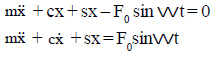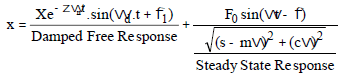TRANSVERSE VIBRATION
Natural frequency of shaft and beams under different type of load and end condition, shows transverse vibration.

•  In case of shafts and beams of negligible mass carrying a concentrated mass, the  force is proportional to the deflection of the mass from the equilibrium position.

Where,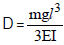for cantilevers supporting a concentrated mass at free end.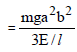for simply supported beams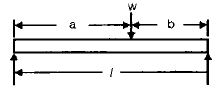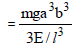for beams fixed at both ends
Note : A shaft supported in long bearings is assumed to have both end fixed while one in short bearing is considered to be simply supported.
(ii) Uniformly loaded Shaft (Simply supported)

•   A simply supported shaft carrying a uniformly distributed mass has maximum  deflection at the mid-span.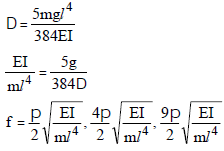Then, taking the smallest value of fn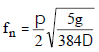Note:

•  This is the lowest frequency of transverse vibrations and is called the fundamental frequency. It has a node at each end.
•  The next higher frequency is four times the fundamental frequency. It has three nodes.
•   The next higher frequency is nine times the fundamental. It has four nodes.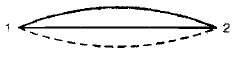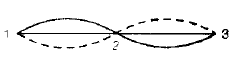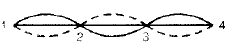•  Thus a simply supported shaft will have an infinite number of frequencies under uniformly distributed load.
•   Similarly, the cases of cantilevers and shafts fixed at both ends can be considered.

A. Dunkerley's Method

•  It is semi-empirical. This gives approximate results but is simple.

fn = frequency of transverse vibration of the whole system
fns = frequency with the distributed load acting alone.
fn1, fn2, fn3 ....... = frequencies of transverse vibrations when each of w1, w2, w3
.... alone.
Then, according do Dunkerley's empirical formula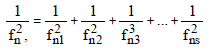where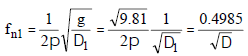Similarly,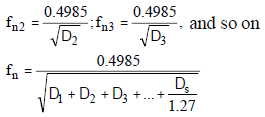B. Energy Method

•  Which gives accurate results but involves heavy calculations if there are many  loads.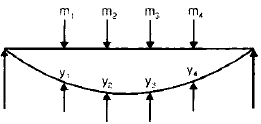Maximum P.E.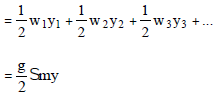Maximum K.E.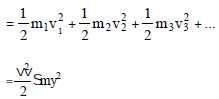ω = circular frequency of vibration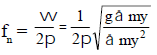WHIRLING OF SHAFT

• When a rotor is mounted on a shaft, its centre of mass does not usually coincide  with the centre line of the shaft. Therefore, when the shaft rotates, it is subjected to  a centrifugal force which makes the shaft bend in the direction of eccentricity of the  centre of mass. Thus further increases the eccentricity, and hence centrifugal force.  The bending of shaft depends upon the eccentricity of the centre of mass of the  rotor as well as upon the speed at which the shaft rotates.
•  Critical or whirling or whipping speed is the speed at which the shaft tends to  vibrate violently in the transverse direction .
The document Vibrations | Mechanical Engineering SSC JE (Technical) is a part of the Mechanical Engineering Course Mechanical Engineering SSC JE (Technical).
All you need of Mechanical Engineering at this link: Mechanical Engineering

## Mechanical Engineering SSC JE (Technical)

6 videos|97 docs|57 tests

## FAQs on Vibrations - Mechanical Engineering SSC JE (Technical)

 1. What is vibration in mechanical engineering?Ans. Vibration in mechanical engineering refers to the periodic motion of an object or system about an equilibrium position. It is caused by the oscillation of particles or components and can be characterized by its frequency, amplitude, and phase.
 2. How does vibration affect mechanical systems?Ans. Vibration can have both positive and negative effects on mechanical systems. On one hand, it can be used for various applications such as vibrating screens, compactors, and vibratory feeders. On the other hand, excessive vibration can lead to fatigue failure, reduced accuracy, increased wear, and decreased lifespan of mechanical components.
 3. What are the common sources of vibration in mechanical systems?Ans. Common sources of vibration in mechanical systems include unbalanced rotating machinery, misalignment, worn-out bearings, resonant frequencies, external forces, and structural vibrations. These sources can generate vibrations that may need to be controlled or eliminated to ensure optimal system performance.
 4. How is vibration measured and analyzed in mechanical engineering?Ans. Vibration is measured using sensors such as accelerometers, displacement transducers, and velocity transducers. These sensors provide data on the amplitude, frequency, and phase of the vibration. The collected data is then analyzed using techniques like Fast Fourier Transform (FFT) to identify the dominant frequencies and modes of vibration.
 5. How can vibration in mechanical systems be controlled or reduced?Ans. Vibration in mechanical systems can be controlled or reduced through various methods. These include balancing rotating components, improving alignment, using damping materials or devices, modifying structural design, implementing vibration isolation techniques, and conducting regular maintenance and inspections to detect and address potential vibration issues.

## Mechanical Engineering SSC JE (Technical)

6 videos|97 docs|57 testsExplore Courses for Mechanical Engineering examSignup to see your scores go up within 7 days! Learn & Practice with 1000+ FREE Notes, Videos & Tests.
10M+ students study on EduRev
Track your progress, build streaks, highlight & save important lessons and more!
Related Searches

,

,

,

,

,

,

,

,

,

,

,

,

,

,

,

,

,

,

,

,

,

;